Ex 9.1

Chapter 9 Class 8 Mensuration
Serial order wise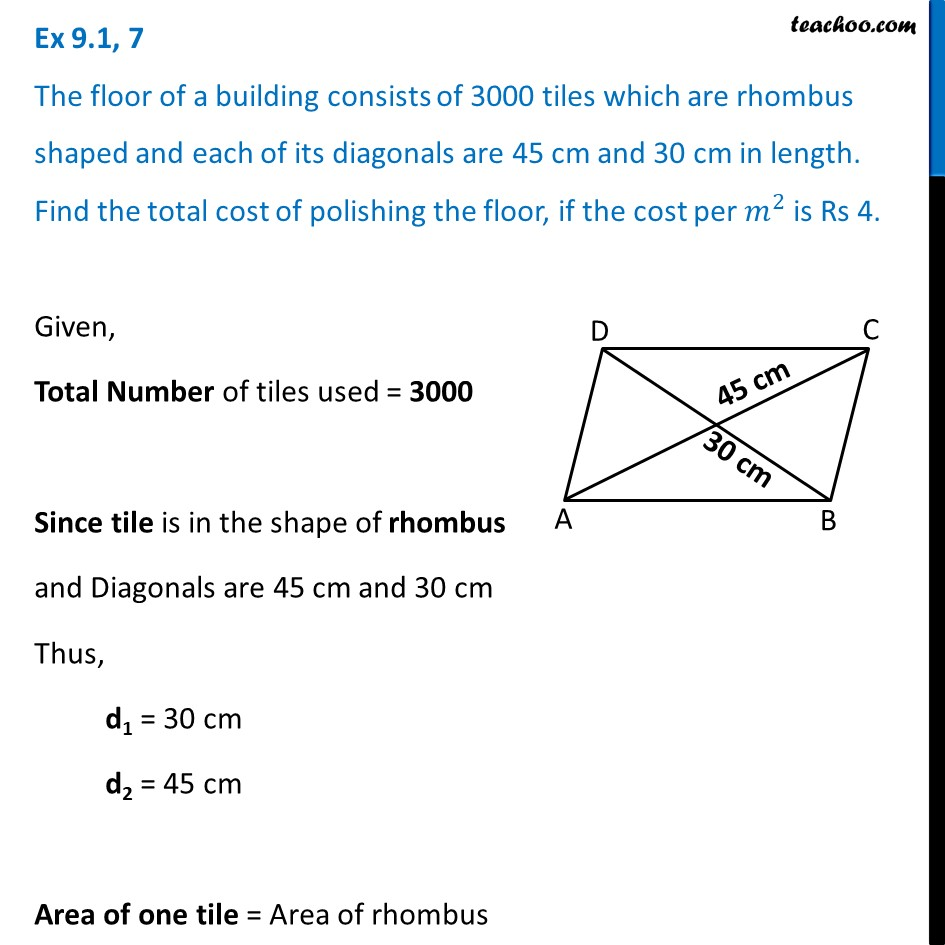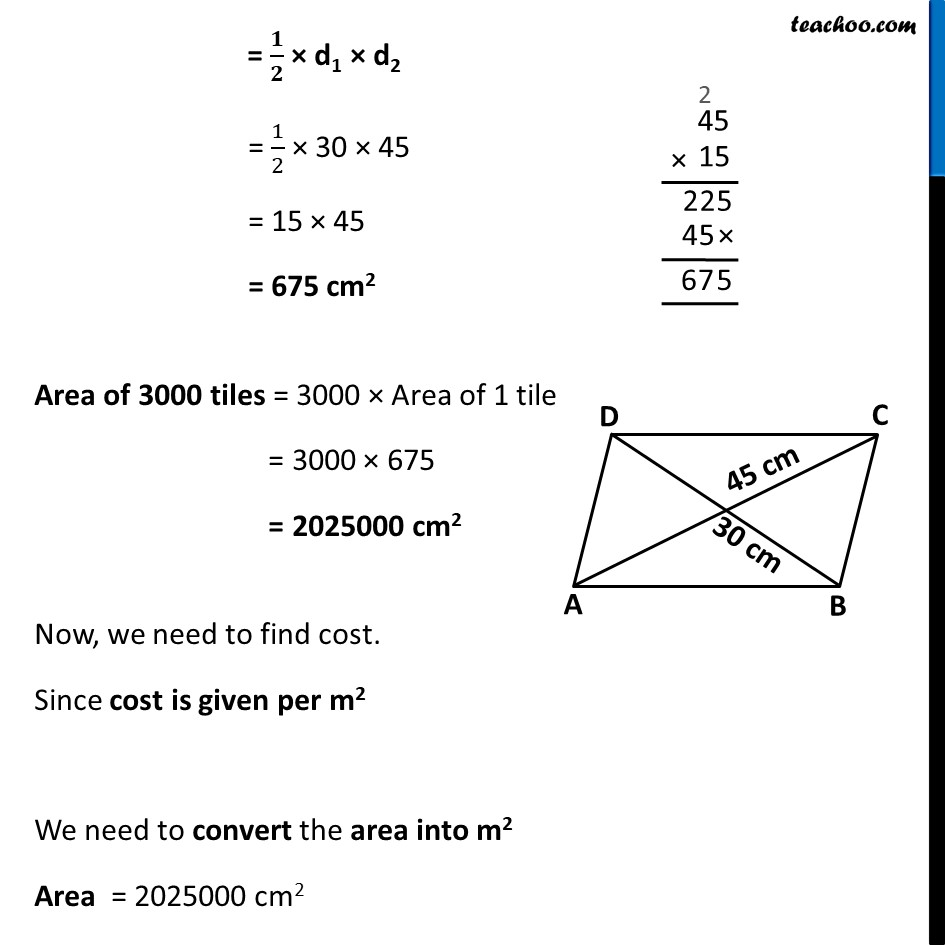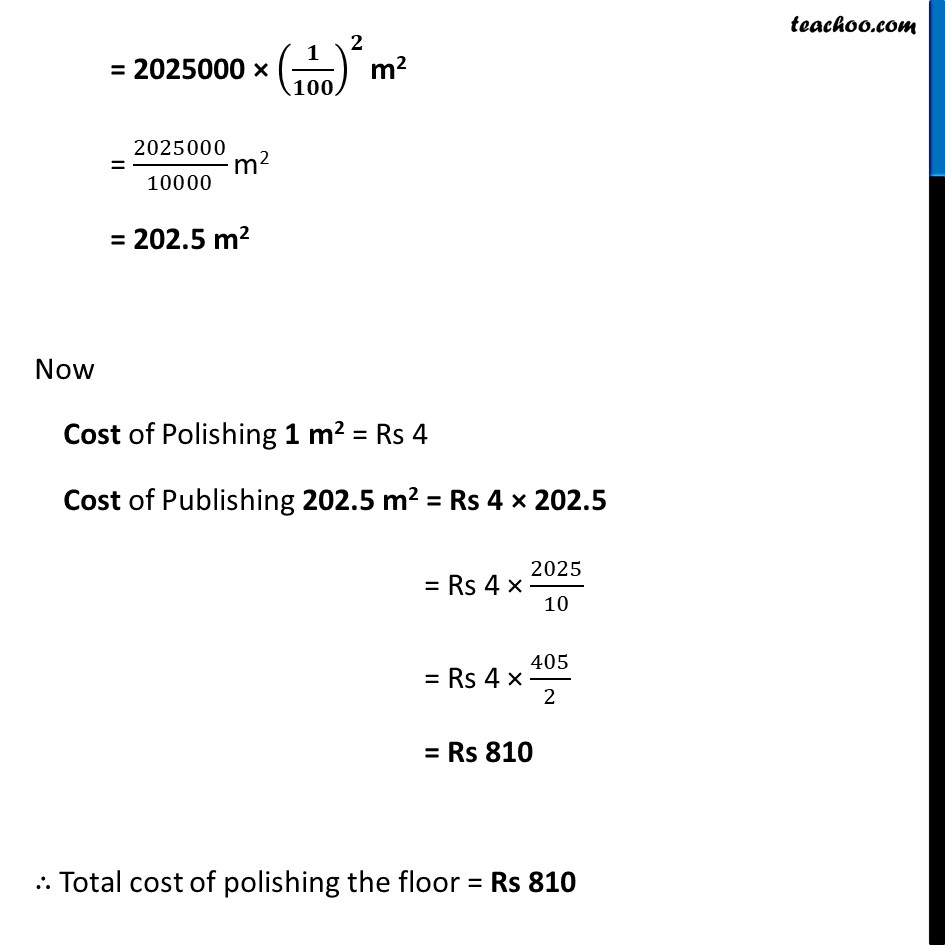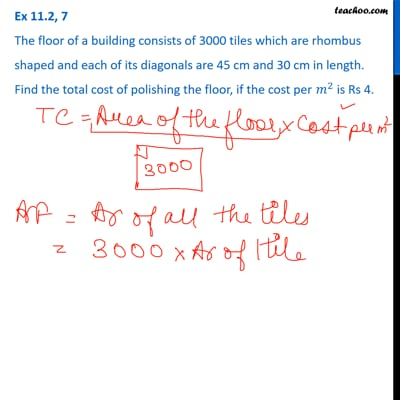This video is only available for Teachoo black users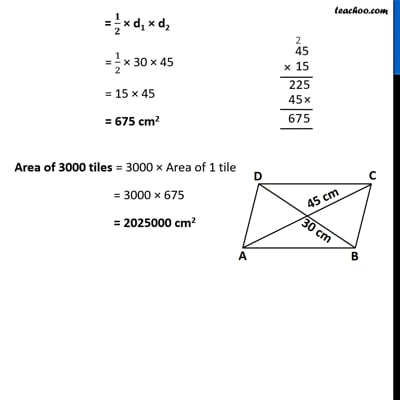This video is only available for Teachoo black users

Learn in your speed, with individual attention - Teachoo Maths 1-on-1 Class

### Transcript

Ex 9.1, 7 The floor of a building consists of 3000 tiles which are rhombus shaped and each of its diagonals are 45 cm and 30 cm in length. Find the total cost of polishing the floor, if the cost per 𝑚^2 is Rs 4.Given, Total Number of tiles used = 3000 Since tile is in the shape of rhombus and Diagonals are 45 cm and 30 cm Thus, d1 = 30 cm d2 = 45 cm Area of one tile = Area of rhombus = 𝟏/𝟐 × d1 × d2 = 1/2 × 30 × 45 = 15 × 45 = 675 cm2 Area of 3000 tiles = 3000 × Area of 1 tile = 3000 × 675 = 2025000 cm2 Now, we need to find cost. Since cost is given per m2 We need to convert the area into m2 Area = 2025000 cm2 = 2025000 × (𝟏/𝟏𝟎𝟎)^𝟐 m2 = 2025000/10000 m2 = 202.5 m2 Now Cost of Polishing 1 m2 = Rs 4 Cost of Publishing 202.5 m2 = Rs 4 × 202.5 = Rs 4 × 2025/10 = Rs 4 × 405/2 = Rs 810 ∴ Total cost of polishing the floor = Rs 810